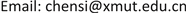1. 引言

2. 相关工作

2.1. 基于产生式学习的在线目标跟踪算法

2.2. 基于产生式学习的在线目标跟踪算法

Babenko等  引入多示例学习到在线目标跟踪里，利用正负包来进行学习。传统的基于MIL的目标跟踪算法  在选择正负包时常用的做法如下：选择当前跟踪器定位的目标位置的样本加入正包，以及从该位置的很近距离范围内抽取一些样本也作为正包中的样本。并从与目标位置有一定距离的位置抽取大量样本构成负包。该做法存在着一些问题，例如当跟踪定位的目标位置不精确时，会导致出现目标漂移。而多数情况下，当选择负包的距离参数设置不准确时，负包中还可能含有部分正样本，从而导致降低跟踪的准确性和鲁棒性。Zhang等人  提出了一种新的在线加权MIL跟踪器(WMIL)。通过样本距离目标位置的远近距离设置相应的权重。而且，算法通过泰勒展开，使用近似计算的方法避免每次选择每个分类器都需做M次概率计算的问题，提高了算法计算效率，从而得到一个更加鲁棒和更快的跟踪器。

3. 基于相关相似度的在线多示例学习目标跟踪算法

3.1. 在线多示例学习框架

3.2. 相关相似度

S ( x i j , x i k ) = 0.5 ( N C C ( x i j , x i k ) + 1 ) (1)

① 正样本最近邻相似度，也叫正最近邻相似度，

S + ( x i j , E ) = max x ∈ 1 j E S ( x i j , x 1 j ) (2)

② 负样本最近邻相似度，也叫负最近邻相似度，

S − ( x 11 , E ) = max x 0 j ∈ E S ( x 11 , x 0 j ) (3)

③ 相关相似度定义如下：

S r ( x 11 , E ) = S + S + + S − (4)

p ( y = 1 | X 1 ) = ∑ j = 1 N 1 w 1 j p ( y 1 = 1 | x 1 j ) (5)

p ( y = 0 | X 0 ) = ∑ j = 1 N 0 w ( 1 − p ( y 0 = 1 | x 0 j ) ) (6)

3.3. 本文算法

SMILE算法的具体算法流程如下：

1：选取一组图像块 X s = { x : ‖ d ( x ) − d t * ‖ < s } 并计算特征向量。

2：使用SMILE分类器来对 x ∈ X s 估计 p ( y | x ) 。

3：更新当前帧的目标位置 d t * = d ( arg max p ( y | x ) ) 。

4：选取两组正包 X 1 = { x : ‖ d ( x ) − d t * ‖ < γ } 和负包 X 0 = { x : γ < ‖ d ( x ) − d t * ‖ < β } 。

5：将包 X i 中每一个示例进行归一化，任意两个图像块 x i j 、 x i k 的相似度被计算为： S ( x i j , x i k ) = 0.5 ( N C C ( x i j , x i k ) + 1 ) 。

6：计算正包中每个示例的正样本最近邻相似度， S + ( x 1 j , E ) = max x 1 k ∈ E S ( x 1 j , x 1 k ) ，以及负样本最近邻相似度， S − ( x 1 j , E ) = max x 0 k ∈ E S ( x 1 j , x 0 k ) 。

7：计算正包中每个示例的相关相似度 S r ( x 1 j , E ) = S + S + + S − 。

8：如果 S r ( x 1 j , E ) ≥ θ ，则图像块 x 1 j 被筛选进新的正包，并计算它的特征向量。

9：利用筛选后的正包、负包中的示例更新弱分类器。

10：计算正包中每个样本的权重 w 1 j = S r ( x 1 j , E ) 。

11：计算示例概率 P ( y i | x i j ) = σ ( H ( x i j ) ) = 1 1 + e − H ( x i j ) 。

12：根据公式(5)和公式(6)计算加权的包概率。

13：得到 L = ∑ i ( log p ( y i | X i ) ) (包的对数似然函数)，通过对它求最大值得到最优的弱分类器， h k * = arg max L ( H k − 1 + h ) 。

14：重复步骤11~13，直到选出的K个最优的弱分类器依次加入当前强分类器，即 H k ( x i j ) = H k − 1 ( x i j ) + h k * 。

4. 实验

The information about the seven video sequence

CarScale250640 × 27242 × 26快速运动，比例变化，遮挡，平面内旋转
Crossing100360 × 24017 × 50比例变化，变形，平面外旋转，快速运动
David3250640 × 48035 × 131遮挡，变形，平面外旋转
Deer60704 × 40095 × 65快速运动，运动模糊，平面内旋转
Dog1950320 × 24051 × 36比例变化，平面外旋转，平面内旋转
Doll1500400 × 30032 × 73光照变化，遮挡，平面内旋转，平面外旋转
FaceOcc1500352 × 288144 × 162遮挡

4.1. 参数设置

4.2. 定量分析4.2.1. 中心位置误差

CLE ( i ) = 1 T ∑ t = 1 T dist ( center i ( i ) , gt ( i ) ) (7)

Mean center location errors (in pixels) for the six trackers and our algorithm. Bold fonts indicate the best performance while italic fonts indicate the second bes

CarScale80.7040.9725.63183.2437.7418.87
Crossing8.416.044.084.1411.443.72
David354.0191.0889.25232.5967.4022.30
Deer15.3116.50240.20117.8216.9210.04
Dog13.7427.347.2761.734.905.87
Doll9.6113.4714.55179.368.2211.14
FaceOcc111.0846.4518.1335.6424.9419.95

4.2.2. 跟踪精度

precision ( τ ) = 1 T ∑ t = 1 T [ 1 R ∑ r = 1 R δ ( dist ( center r ( i ) , gt ( i ) ) ≤ τ ) ] (8)

4.3. 定性分析

5. 结论